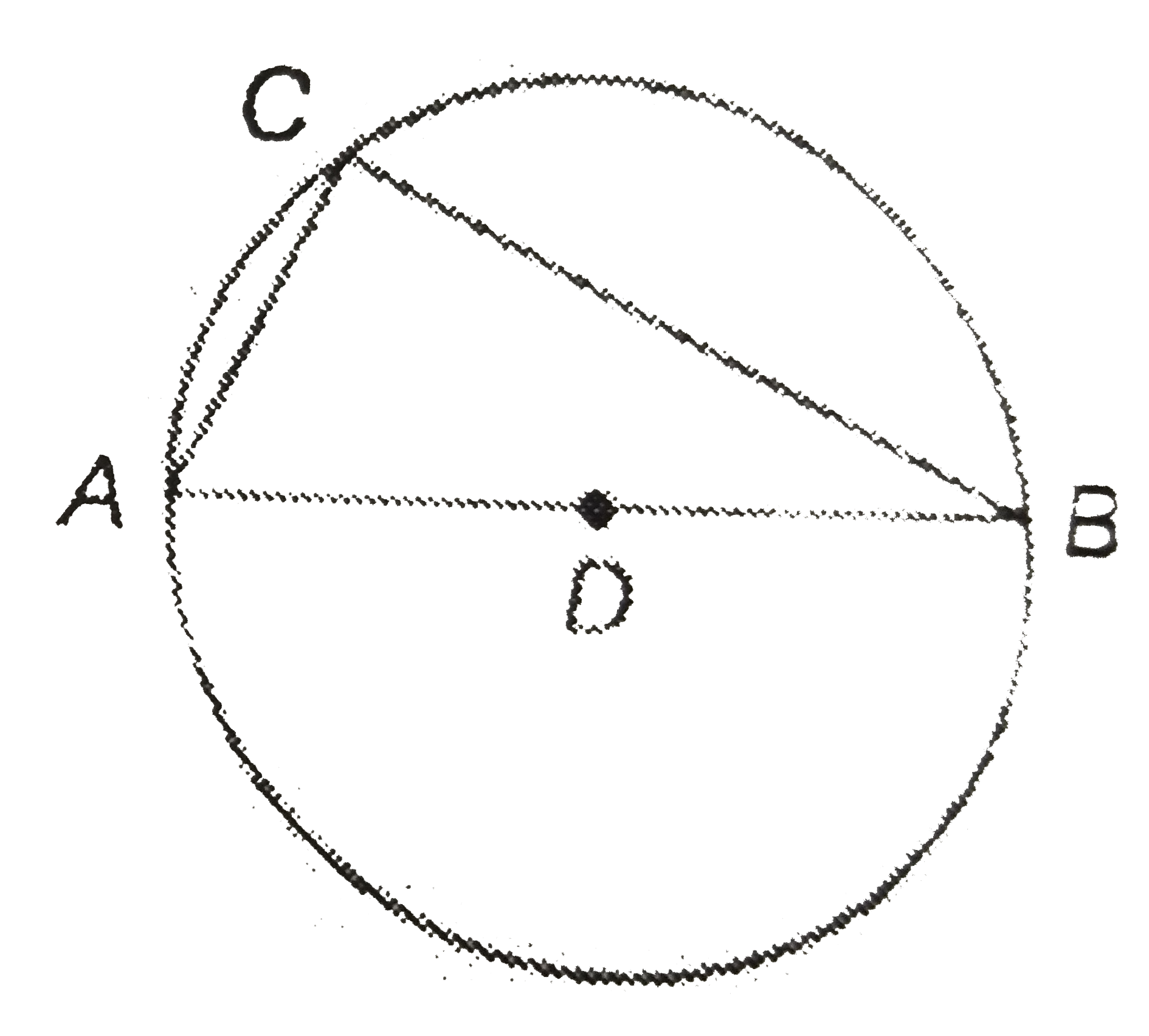# Show that any angle in a semi-circle is a right angle. The following arc the steps involved in showing the above result. Arrange them in sequential or

51 views
in Geometry
closed
Show that any angle in a semi-circle is a right angle.
The following arc the steps involved in showing the above result. Arrange them in sequential order.
A) therefore angleACB=180^(@)/2=90^(@)
B) The angle subtended by an arc at the center is double of the angle subtended by the same arc at any point on the remaining part of the circle.
c) Let AB be a diameter of a circle with center D and C be any point on the circle. Join AC and BC.
D) therefore angleAD = 2 xx angleACB
180^(@)=2angleACB(therefore angleADB=180^(@))A. DBAC
B. DBCA
D. CBDA

by (88.7k points)
selected by

Correct Answer - D
CBDA is the required sequential order.